The LOESS Procedure

Automatic Smoothing Parameter Selection

There are several methodologies for automatic smoothing parameter selection. One class of methods chooses the smoothing parameter value to minimize a criterion that incorporates both the tightness of the fit and model complexity. Such a criterion can usually be written as a function of the error mean square,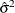, and a penalty function designed to decrease with increasing smoothness of the fit. This penalty function is usually defined in terms of the smoothing matrix(see the section Smoothing Matrix).

Examples of specific criteria are generalized cross validation (Craven and Wahba, 1979) and the Akaike information criterion (Akaike, 1973). These classical selectors have two undesirable properties when used with local polynomial and kernel estimators: they tend to undersmooth small data sets and tend to be nonrobust in the sense that small variations of the input data can change the choice of smoothing parameter value significantly. Hurvich, Simonoff, and Tsai (1998) obtained several corrected AIC criteria that address the small-sample bias and perform comparably with the plug-in selectors (Ruppert, Sheather, and Wand, 1995). PROC LOESS provides automatic smoothing parameter selection that uses two of these corrected AIC criteria, named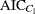and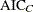in Hurvich, Simonoff, and Tsai (1998), and generalized cross validation, denoted by GCV.

The relevant formulas are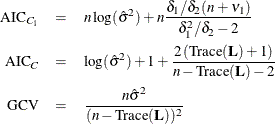where n is the number of observations and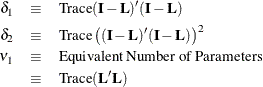You invoke these methods for automatic smoothing parameter selection by specifying the SELECT=criterion option in the MODEL statement, where criterion is AICC1, AICC, or GCV. The LOESS procedure evaluates the specified criterion for a sequence of smoothing parameter values and selects the value in this sequence that minimizes the specified criterion. If multiple values yield the optimum, then the largest of these values is selected.

A second class of methods seeks to set an approximate measure of model degrees of freedom to a specified target value. These methods are useful for making meaningful comparisons between loess fits and other nonparametric and parametric fits. Three approximate model degrees of freedom for a loess model are defined in the section Model Degrees of Freedom. You invoke these methods by specifying the SELECT=DFCriterion(target) option in the MODEL statement, where DFCriterion is DF1, DF2, or DF3. The criterion that is minimized is given in the following table.

Table 59.4: Minimization Criteria

Syntax

Minimization Criterion

SELECT=DF1(target)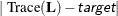SELECT=DF2(target)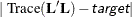SELECT=DF3(target)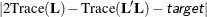The results are summarized in the "Smoothing Criterion" table. This table is displayed whenever automatic smoothing parameter selection is performed. You can obtain details of the sequence of models examined by specifying the DETAILS(MODELSUMMARY) option in the MODEL statement to display the "Model Summary" table.

There are several ways in which you can control the sequence of models examined by PROC LOESS. If you specify the SMOOTH=value-list option in the MODEL statement, then only the values in this list are examined in performing the selection. For example, the following statements select the model that minimizes the AICC1 criterion among the three models with smoothing parameter values 0.1, 0.3, and 0.4:

proc loess;
model y= x1/ smooth=0.1 0.3 0.4 select=AICC1;
run;

If you do not specify the SMOOTH= option in the MODEL statement, then by default PROC LOESS uses a golden section search method to find a local minimum of the specified criterion in the range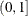. You can use the RANGE(lower,upper) modifier in the SELECT= option to change the interval in which the golden section search is performed. For example, the following statements request a golden section search to find a local minimizer of the GCV criterion for smoothing parameter values in the interval [0.1,0.5]:

proc loess;
model y= x1/select=GCV( range(0.1,0.5) );
run;

If you want to be sure of obtaining a global minimum in the range of smoothing parameter values examined, you can specify the GLOBAL modifier in the SELECT= option. For example, the following statements request that a global minimizer of the AICC criterion be obtained for smoothing parameter values in the interval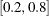:

proc loess;
model y= x1/select=AICC( global range(0.2,0.8) );
run;

Note that even though the smoothing parameter is a continuous variable, a given range of smoothing parameter values corresponds to a finite set of local models. For example, for a data set with 100 observations, the rangecorresponds to models with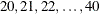points in the local neighborhoods. If the GLOBAL modifier is specified, all possible models in the range are evaluated sequentially.

Note that by default PROC LOESS displays a "Fit Summary" and other optionally requested tables only for the selected model. You can request that these tables be displayed for all models in the selection process by adding the STEPS modifier in the SELECT= option. Also note that by default scoring requested with SCORE statements is done only for the selected model. However, if you specify the STEPS in both the MODEL and SCORE statements, then all models evaluated in the selection process are scored.

In terms of computation,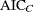, GCV, and DF1 depend on the smoothing matrixonly through its trace. In the direct method, this trace can be computed efficiently. In the interpolated method that uses k-d trees, there is some additional computational cost but the overall work is not significant compared to the rest of the computation. In contrast, the quantities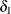,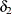, and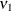that appear in thecriterion, and the DF2 and DF3 criteria, depend on the entirematrix and for this reason, the time needed to compute these quantities dominates the time required for the model fitting. Hence SELECT=AICC1, SELECT=DF2, and SELECT=DF3 are much more computationally expensive than SELECT=AICC, SELECT=GCV, and SELECT=DF1, especially when combined with the GLOBAL modifier. Hurvich, Simonoff, and Tsai (1998) note thatcan be regarded as an approximation ofand that "theselector generally performs well in all circumstances."

For models with one dependent variable, PROC LOESS uses SELECT=AICC as its default, if you specify neither the SMOOTH= nor the SELECT= option in the MODEL statement. With two or more dependent variables, automatic smoothing parameter selection needs to be done separately for each dependent variable. For this reason automatic smoothing parameter selection is not available for models with multiple dependent variables. In such cases you should use a separate PROC LOESS step for each dependent variable, if you want to use automatic smoothing parameter selection.